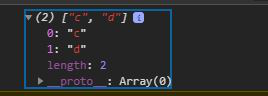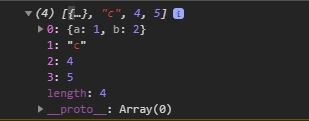# Ramda.js R.without() Function

• Last Updated : 08 Jul, 2020

Ramda.js is a library in JavaScript that is used for playing with array, strings, objects etc, but in a functional way. The without() function in Ramda is used to return the array with the particular set of values removed from it. It works as filter in javascript.

Syntax:

`R.without(elementArray, array)`

Parameters: This function accept two parameters as mentioned above and described below:

• elementArray: It is the array of elements to be removed from the original array.
• array: It is the original array to be given as second parameter.

Note: Both parameters are necessary to be given.

Return Value: The return type of this function is array .

Below examples illustrate the R.without() function in Ramda.js.

Example 1:

## HTML

 ```<``html` `lang``=``"en"``>`` ` `<``head``>``    ``<``meta` `charset``=``"UTF-8"``>``    ``<``meta` `name``=``"viewport"` `content``=``        ``"width=device-width, initial-scale=1.0"``>``    ``<``title``>NodeJS Ramda.replace() method```` ` `<``body``>``    ````    ``<``script` `src``=``"//cdnjs.cloudflare.com/ajax/libs/ramda/0.25.0/ramda.min.js"``>``    ````     ` `    ``<``script``>``        ``let elementArray = ["a", "b"];``        ``let array = ["a", "a", "b", "c", "d"]`` ` `         ``// Applying R.without function``        ``const newArray = R.without(elementArray, array);``        ``console.log(newArray)``    `````` ` ``

Output:Example 2: When an array of objects is given and array is filtered by the array of object.

## Javascript

 ````` ` ```    ````    ````    ``NodeJS Ramda.replace() ``function`````` ` ```    ````    ````     ` `    `````` ` ``

Output: